# Common Core: 1st Grade Math : Understanding Place Value

## Example Questions

### Example Question #455 : Whole And Part

What digit is in the ones place?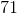NeitherExplanation:

The ones place is always the first number on the right.

### Example Question #456 : Whole And Part

What digit is in the ones place?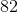NeitherExplanation:

The ones place is always the first number on the right.

### Example Question #11 : Understanding Place Value

What digit is in the ones place?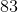Explanation:

The ones place is always the first number on the right.

### Example Question #12 : Understanding Place Value

What digit is in the ones place?Explanation:

The ones place is always the first number on the right.

### Example Question #13 : Understanding Place Value

What digit is in the ones place?Explanation:

The ones place is always the first number on the right.

### Example Question #14 : Understanding Place Value

What digit is in the ones place?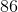Explanation:

The ones place is always the first number on the right.

### Example Question #992 : Common Core Math: Grade 1

What digit is in the ones place?Explanation:

The ones place is always the first number on the right.

### Example Question #15 : Understanding Place Value

What digit is in the ones place?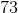Explanation:

The ones place is always the first number on the right.

### Example Question #16 : Understanding Place Value

What digit is in the ones place?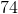Explanation:

The ones place is always the first number on the right.

### Example Question #17 : Understanding Place Value

What digit is in the ones place?